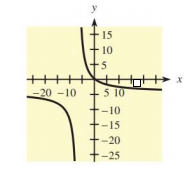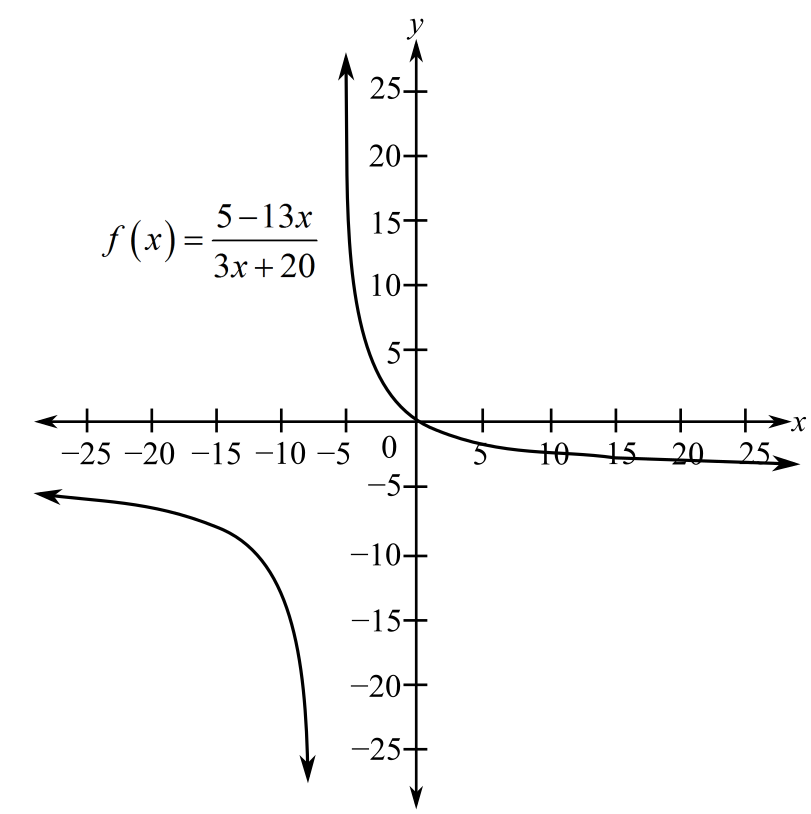Chapter 10.5, Problem 26E### Mathematical Applications for the ...

11th Edition
Ronald J. Harshbarger + 1 other
ISBN: 9781305108042

#### Solutions

Chapter
Section### Mathematical Applications for the ...

11th Edition
Ronald J. Harshbarger + 1 other
ISBN: 9781305108042
Textbook Problem

# In Problems 25-28, a function and its graph are given.(a) Use the graph to estimate the locations of any horizontal or vertical asymptotes.(b) Use the function to determine precisely the locations of any asymptotes. f ( x ) = 5 − 13 x 3 x + 20(a)

To determine

The vertical and horizontal asymptotes from the graph for the provided graph of the function f(x)=513x3x+20.Explanation

Given Information:

The provided graph of the function f(x)=513x3x+20 is

.

Explanation:

Consider the provided graph,

Recall that a vertical asymptote of a function f(x) is a line x=a such that f(a)=.

At x5 the graph of the function decreases and increases infinitely.

Thus, the vertical asymptote is the line x5

(b)

To determine

To calculate: The vertical and horizontal asymptotes for the function f(x)=513x3x+20.

### Still sussing out bartleby?

Check out a sample textbook solution.

See a sample solution

#### The Solution to Your Study Problems

Bartleby provides explanations to thousands of textbook problems written by our experts, many with advanced degrees!

Get Started

#### Find more solutions based on key concepts